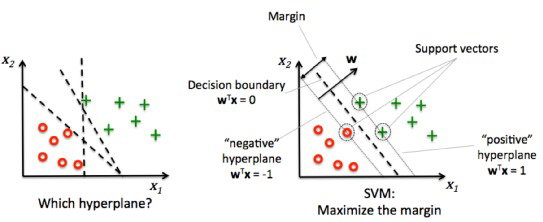# Data Science Algorithms: SVM

18 Sep
18Sep

## What is Support Vector Machine?

“Support Vector Machine” (SVM) is a supervised machine learning algorithm which can be used for both classification or regression challenges. However,  it is mostly used in classification problems. In this algorithm, we plot each data item as a point in n-dimensional space (where n is number of features you have) with the value of each feature being the value of a particular coordinate. Then, we perform classification by finding the hyper-plane that differentiate the two classes very well (look at the below snapshot).Support Vectors are simply the co-ordinates of individual observation. Support Vector Machine is a frontier which best segregates the two classes (hyper-plane/ line).

What is Support Vector Machine?

An SVM model is a representation of the examples as points in space, mapped so that the examples of the separate categories are divided by a clear gap that is as wide as possible.
In addition to performing linear classification, SVMs can efficiently perform a non-linear classification, implicitly mapping their inputs into high-dimensional feature spaces.

What does SVM do?

Given a set of training examples, each marked as belonging to one or the other of two categories, an SVM training algorithm builds a model that assigns new examples to one category or the other, making it a non-probabilistic binary linear classifier.

## Pros and Cons associated with SVM

• Pros:
• It works really well with clear margin of separation
• It is effective in high dimensional spaces.
• It is effective in cases where number of dimensions is greater than the number of samples.
• It uses a subset of training points in the decision function (called support vectors), so it is also memory efficient.
• Cons:
• It doesn’t perform well, when we have large data set because the required training time is higher
• It also doesn’t perform very well, when the data set has more noise i.e. target classes are overlapping
• SVM doesn’t directly provide probability estimates, these are calculated using an expensive five-fold cross-validation. It is related SVC method of Python scikit-learn library.Read more

## Support Vector Machine vs Logistic Regression

• SVM try to maximize the margin between the closest support vectors while LR the posterior class probability. Thus, SVM find a solution which is as fare as possible for the two categories while LR has not this property.